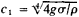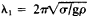Waves on the Surface of a Liquid

The following article is from The Great Soviet Encyclopedia (1979). It might be outdated or ideologically biased.

Waves on the Surface of a Liquid

waves that develop and propagate along the free surface of a liquid or along the interface between two immiscible liquids. Such waves are formed by an external action that disturbs the equilibrium condition of the liquid surface—for example, when a stone is dropped. Forces are thereby produced that reestablish the equilibrum: these are the forces of surface tension and gravity. Depending on the nature of the restoring forces, the waves on the surface of a liquid may be subdivided into capillary waves, where the forces of surface tension predominate, and gravitational waves, where gravitational forces predominate. When both types offerees act together, the waves are called gravitational-capillary. The effect of surface tension is more important at short wavelengths, and the effect of gravity is more important at long wavelengths.

The propagation velocity c of waves on the surface of a liquid is a function of the wavelength λ. As the wavelength increases, the velocity of propagation of gravitational-capillary waves at first decreases to some minimum valueand then increases again (σ is the surface tension, g is the acceleration of gravity, and ρ is the density of the liquid). The value of c1 corresponds to the wavelength. When λ>λ1 the velocity of propagation is primarily a function of the gravitational force, but when λ<λ1, it is mainly dependent on the surface tension. For an air-water interface λ1 = 1.72 cm.

The reasons for the development of gravitational waves are the attraction of the liquid by the sun and moon (tides), the movement of bodies close to or on the surface of the water (ships’ wakes), the action of a system of pressure pulses on the surface of the liquid (wind waves or the initial displacement of some part of the surface from an equilibrium position, such as a local increase in the level of the liquid by an underwater explosion). The most common natural phenomena are wind waves.